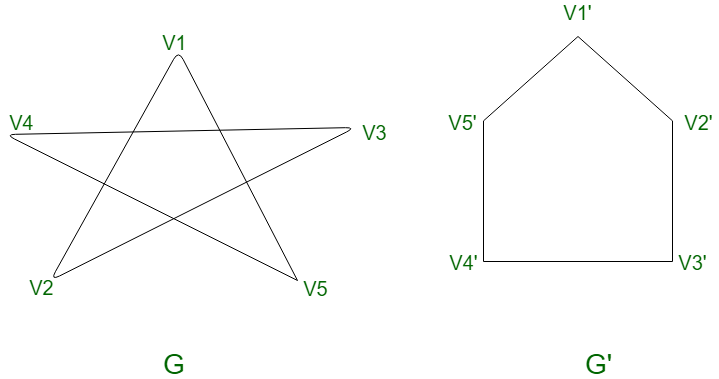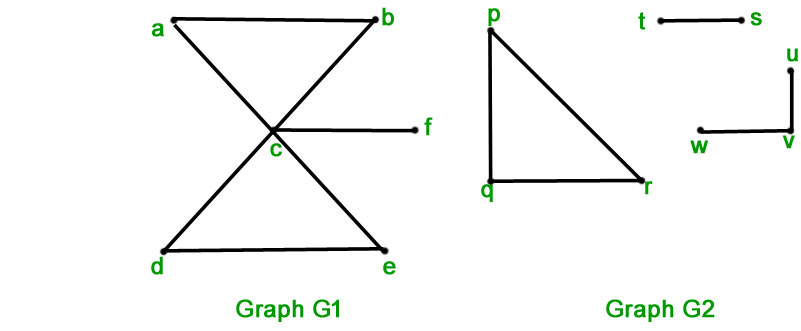Mathematics | Graph Isomorphisms and Connectivity

• Difficulty Level : Medium
• Last Updated : 15 Jun, 2020

Isomorphism :

Consider the following two graphs –Are the graphsandthe same?

If your answer is no, then you need to rethink it. The graphical arrangement of the vertices and edges makes them look different, but they are the same graph. Also notice that the graphis a cycle, specifically. To know about cycle graphs read Graph Theory Basics.

Formally,
“The simple graphsandare isomorphic if there is a bijective functionfromtowith the property thatandare adjacent inif and only ifandare adjacent in.”

Example : Show that the graphsandmentioned above are isomorphic.

Solution : Letbe a bijective function fromto.
Let the correspondence between the graphs be-The above correspondence preserves adjacency as-is adjacent toandin, andis adjacent toandinSimilarly, it can be shown that the adjacency is preserved for all vertices.
Hence,andare isomorphic.

Proving that the above graphs are isomorphic was easy since the graphs were small, but it is often difficult to determine whether two simple graphs are isomorphic. This is because there arepossible bijective functions between the vertex sets of two simple graphs withvertices. Testing the correspondence for each of the functions is impractical for large values of n.
Although sometimes it is not that hard to tell if two graphs are not isomorphic. In order, to prove that the given graphs are not isomorphic, we could find out some property that is characteristic of one graph and not the other. If they were isomorphic then the property would be preserved, but since it is not, the graphs are not isomorphic.
Such a property that is preserved by isomorphism is called graph-invariant.

Some graph-invariants include- the number of vertices, the number of edges, degrees of the vertices, and length of cycle, etc.

You can say given graphs are isomorphic if they have:

1. Equal number of vertices.
2. Equal number of edges.
3. Same degree sequence
4. Same number of circuit of particular length

In most graphs checking first three conditions is enough.

Important Note : The complementary of a graph has the same vertices and has edges between any two vertices if and only if there was no edge between them in the original graph. Consequently, a graphis said to be self-complementary if the graph and its complement are isomorphic.

Connectivity :

Most problems that can be solved by graphs, deal with finding optimal paths, distances, or other similar information. Almost all of these problems involve finding paths between graph nodes.

Path – A path of lengthfromtois a sequence ofedgessuch thatis associated with, and so on, withassociated with, whereand.

Note : A path is called a circuit if it begins and ends at the same vertex. It is also called a cycle.

Connectivity of a graph is an important aspect since it measures the resilience of the graph.
“An undirected graph is said to be connected if there is a path between every pair of distinct vertices of the graph.”

Connected Component – A connected component of a graphis a connected subgraph ofthat is not a proper subgraph of another connected subgraph of.

For example, in the following diagram, graphis connected and graphis disconnected. Sinceis connected there is only one connected component.
But in the case ofthere are three connected components.In case the graph is directed, the notions of connectedness have to be changed a bit. This is because of the directions that the edges have.

Formally,
“A directed graph is said to be strongly connected if there is a path fromtoandtowhereandare vertices in the graph. The graph is weakly connected if the underlying undirected graph is connected.”

Strongly Connected Component –
Analogous to connected components in undirected graphs, a strongly connected component is a subgraph of a directed graph that is not contained within another strongly connected component.

Articulation points –

The removal of a vertex and all the edges incident with it may result in a subgraph that has more connected components than in the original graphs. Such vertices are called articulation points or cut vertices.
Analogous to cut vertices are cut edge the removal of which results in a subgraph with more connected components. A cut-edge is also called a bridge.

Cut set – In a connected graph, a cut-set is a set of edges which when removed fromleavesdisconnected, provided there is no proper subset of these edges disconnects.

Paths and Isomorphisms –

Sometimes even though two graphs are not isomorphic, their graph invariants- number of vertices, number of edges, and degrees of vertices all match. In this case paths and circuits can help differentiate between the graphs.

• Example – Are the two graphs shown below isomorphic?• Solution – Both the graphs have 6 vertices, 9 edges and the degree sequence is the same. However the second graph has a circuit of length 3 and the minimum length of any circuit in the first graph is 4. Hence the given graphs are not isomorphic.

GATE CS Corner Questions

Practicing the following questions will help you test your knowledge. All questions have been asked in GATE in previous years or GATE Mock Tests. It is highly recommended that you practice them.

References-

Graph Isomorphism – Wikipedia
Graph Connectivity – Wikipedia
Discrete Mathematics and its Applications, by Kenneth H Rosen

This article is contributed by Chirag Manwani. If you like GeeksforGeeks and would like to contribute, you can also write an article using contribute.geeksforgeeks.org or mail your article to contribute@geeksforgeeks.org. See your article appearing on the GeeksforGeeks main page and help other Geeks.